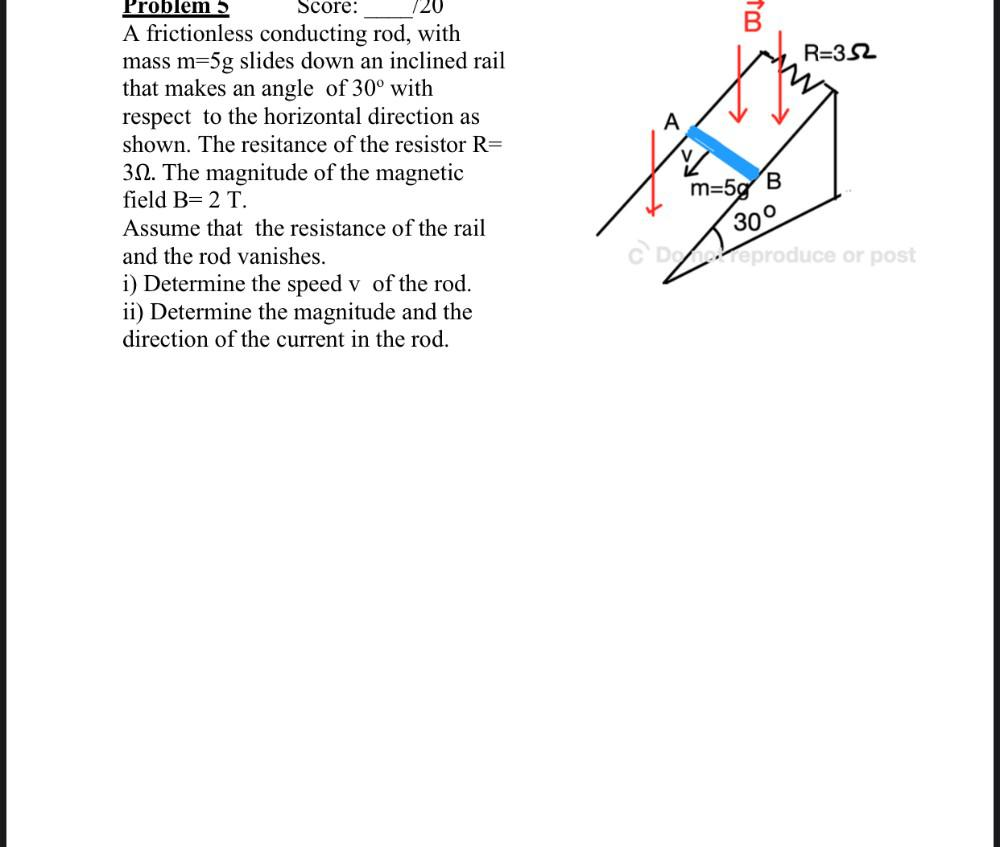Question:

# B R=352 A Problem Score: A frictionless conducting rod, with mass m=5g slides down an inclined rail that makes an angle of 30° wB R=352 A Problem Score: A frictionless conducting rod, with mass m=5g slides down an inclined rail that makes an angle of 30° with respect to the horizontal direction as shown. The resitance of the resistor R= 312. The magnitude of the magnetic field B= 2 T. Assume that the resistance of the rail and the rod vanishes. i) Determine the speed v of the rod. ii) Determine the magnitude and the direction of the current in the rod. m=5g 300 CD Veproduce or post